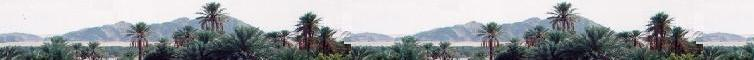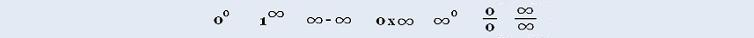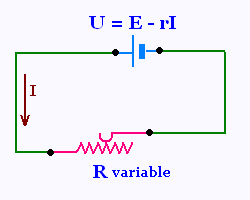###### Calculus I

Exercices

Applications

Marginal analysis

© The scientific sentence. 2010

# Calculus I: Derivative applications Ohmic circuit

##### Ohmic circuitLet's consider an electric circuit including a battery and an exterior variable resistance (resistor) R.

The battery has a constant emf = E, and an interior constant resistance r.

a) What is the value of the resistor R to have a max power in the circuit?

U increases at the rate of 0.5 volt/sec, while I decreases 0.1 amps/sec. t is the time in seconds,

b) find an equation relating dR/dt dV/dt and dI/dt

c) find dR/dt when V = 9 volts and I = 3 A.

We known that U = E - rI and U = IR. Where I is the intensity of the electric current in the circuit, and U is the voltage.

The know also that P = U I = RI2. Where P is the electric power of the circuit. So

U = RI = E - rI. Then

E = (r + R)I or I = E/(r + R)

P = U I = RI2 = R (E/(r + R))2 =
E2 x R/(r + R)2 =

P = E2 R/(r + R)2

The maximum of P is obtained by taking its derivative equal to zero. That is : dP/dR = 0.

dP/dR = E2 ((r + R)2 - 2R(r + R))/(r + R)4 =
E2 (r2 + 2rR + R2 - 2Rr - 2R2)/(r + R)4 =
E2 (r2 - R2)/(r + R)4 =
E2 (r + R)(r - R)/(r + R)4 =
E2(r - R)/(r + R)3

dP/dR = E2(r - R)/(r + R)3

dP/dR = 0 yields r = R. Hence

The power is maximum in the R-circuit when the interior resistance is equal to the exerior resistance.

b) U = RI. Taking the differential yields:

dU/dt = R dI/dt + I dR/dt. Since U = RI, one has

dU/dt = (U/I) dI/dt + I dR/dt

c) dR/dt = (1/I) (dU/dt - (U/I) dI/dt) =
(1/3) (0.5 - (9/3) x 0.1) = (0.5 - 0.3)/3 = 0.067.

dR/dt = 0.067 Ω/sec.

dR/dt = 0.067 Ω/sec.

 chimie labs | Physics and Measurements | Probability & Statistics | Combinatorics - Probability | Chimie | Optics | contact |